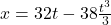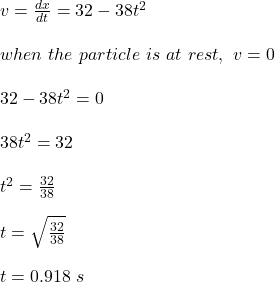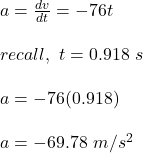Question

A particle moves along a straight line. Its position at any instant is given by x = 32t− 38t^3/3 where x is in metre and t in second. Find the acceleration of the particle at the instant when particle is at rest.

1.kimcuc

The acceleration of the object is -69.78 m/s²

Explanation:

Given;

postion of the particle:The velocity of the particle is calculated as the change in the position of the  particle with time;Acceleration is the change in velocity with time;